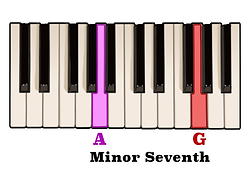top of page

## Seventh Chords Construction | Fully Diminished Seventh Chords (5 of 5)

A fully diminished seventh chord consists of a diminished triad with an interval of a diminished seventh above the root note.

As we learned in the previous video a diminished triad is essentially a minor triad with a flattened fifth.Therefore, if the notes of an A minor triad are A, C, and E,then the notes of an A diminished triad would be A, C, and E-flat.

Now, an interval of a diminished seventh can be thought of as being a flattened minor seventh interval.In other words, if an interval of a minor seventh above the note A is the note G,then an interval of a diminished seventh above the note A is the note G-flat.

By playing the notes of an A diminished triad together with an interval of a diminished seventh above the note A we will have constructed an A fully diminished seventh chord.Likewise, the notes of a B diminished triad are B, D, and F.If an interval of a minor seventh above B is the note A, then an interval of a diminished seventh above B would be the note A-flat.Adding the note A-flat to the notes of a B diminished triad would result in a B fully diminished seventh.

Another way to think about a fully diminished seventh chord is by the intervals which separate each consecutive note.

Fully diminished seventh chords are unique in that each note of the chord is separated by an interval of a minor third.With this in mind, let's construct a fully diminished seventh chord above the note E.An interval of a minor third above the note E is the note G. Another interval of a minor third above the note G is the note B-flat And an interval of a minor third above the note B-flat is the note D-flat.

Here we can see that the notes of an E fully diminished seventh are E, G, B-flat, and D-flat. By following this pattern of intervals you will be able to construct a fully diminished seventh chord above any root note.Now, because the notes of a fully diminished seventh chord are separated by an interval of a minor third, each note is equidistant from the next. Therefore, it does not matter which inversion the chord is in -  each consecutive note will always be separated by three consecutive half steps.

bottom of page